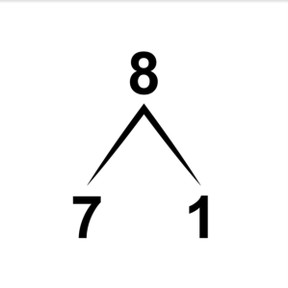Decomposing and composing number to 10

# Decomposing and composing number to 10

Decomposing and composing number to 10

No account needed.8,000 schools use Gynzy92,000 teachers use Gynzy1,600,000 students use Gynzy

## General

Students learn to decompose and compose the numbers to 10. They learn to recognize decompositions in color, but also using number bonds and arrows. They learn to compose numbers by counting on.

## Relevance

It is useful to know how to split something up in different ways. You can divide 4 cookies into 4 and 0, but also in 2 and 2. Ask students to come up with other decompositions.

## Introduction

Students practice counting to 10 by counting the different balls in the picture.

## Development

You can decompose numbers in different ways. Using the fish, demonstrate that you can decompose the number 6 into 3 and 3, but also in 4 and 2. This is called decomposing a number. If you already have one of the two numbers, you can count on to the given number. This is called composing a number. Show decomposition by dividing the fish between the two fishbowls. Then show all possible decompositions of 7. Explain that sometimes you can already see the decomposition in an image, by color for example. Also mention that you can switch numbers in a number bond. Discuss that you can use an arrow to write down the number bonds. Practice recognizing numbers on a bead string and discuss which numbers can be switched and how to write them with an arrow. Explain to students that if you can't see the decomposition, you need to count the total and then count on to the given number. Practice together and check that students can compose numbers. There are also decompositions where you get a number without any visual support. Ask students to try to decompose the number in their heads, but say that they may use blocks to support their learning.

To check that students can decompose and compose numbers to 10 you can ask the following questions:
- What does decomposing numbers mean? And composing numbers?
- How can you check if a decomposition is correct? (count total)
- Can you give two examples of decompositions of 6? And of other numbers?

## Guided Practice

Students practice decomposition by first working with number bonds with one number already given. Then they are given objects to count, and finally students are asked to decompose numbers in their heads.

## Closing

Check that students can decompose and compose numbers to 10 by asking them to fill in the missing numbers. Discuss that it is useful to be able to decompose so you know different ways to split a total. Ask students to name other examples of this.

## Teaching Tip

Students who have difficulty decomposing and composing can be supported by using manipulatives while working with the numbers.

## Instruction materials

blocks

### The online teaching platform for interactive whiteboards and displays in schools

• Save time building lessons

• Manage the classroom more efficiently

• Increase student engagement